Next: Ion Sound Waves Up: Waves in Warm Plasmas Previous: Physics of Landau Damping

# Plasma Dispersion Function

If the unperturbed distribution function,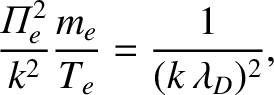, appearing in Eq. (1007), is a Maxwellian then it is readily seen that, with a suitable scaling of the variables, the dispersion relation for electrostatic plasma waves can be expressed in terms of the function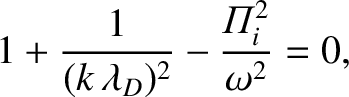(1022)

which is defined as it is written for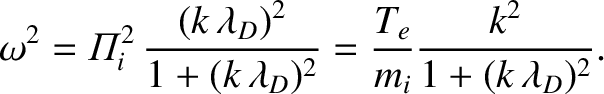, and is analytically continued for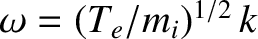. This function is known as the plasma dispersion function, and very often crops up in problems involving small-amplitude waves propagating through warm plasmas. Incidentally,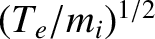is the Hilbert transform of a Gaussian.

In view of the importance of the plasma dispersion function, and its regular occurrence in the literature of plasma physics, let us briefly examine its main properties. We first of all note that if we differentiatewith respect to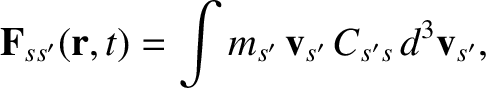we obtain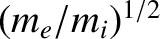(1023)

which yields, on integration by parts,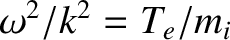(1024)

If we lettend to zero from the upper half of the complex plane, we get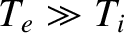(1025)

Note that the principle part integral is zero because its integrand is an odd function of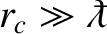.

Integrating the linear differential equation (1024), which possesses an integrating factor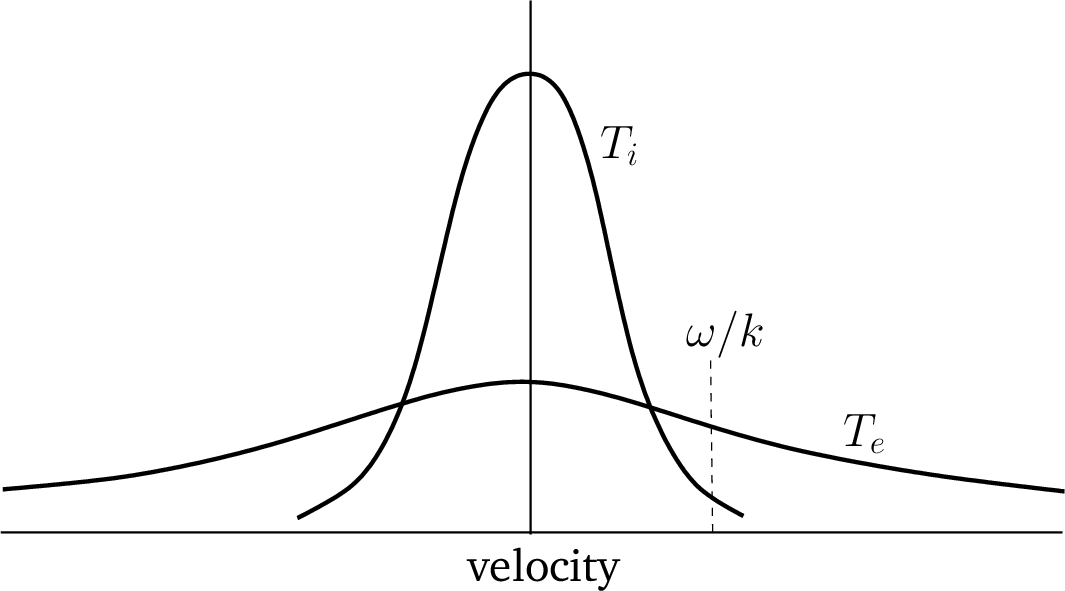, and using the boundary condition (1025), we obtain an alternative expression for the plasma dispersion function: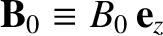(1026)

Making the substitution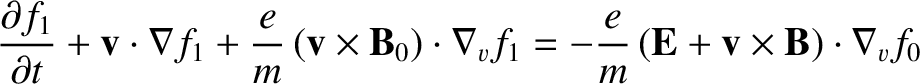in the integral, and noting that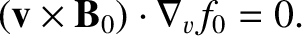(1027)

we finally arrive at the expression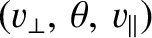(1028)

This formula, which relates the plasma dispersion function to an error function of imaginary argument, is valid for all values of.

For smallwe have the expansion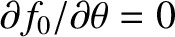(1029)

For large, where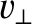, the asymptotic expansion foris written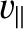(1030)

Here,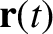(1031)

In deriving our expression for the Landau damping rate we have, in effect, used the first few terms of the above asymptotic expansion.

The properties of the plasma dispersion function are specified in exhaustive detail in a well-known book by Fried and Conte.Next: Ion Sound Waves Up: Waves in Warm Plasmas Previous: Physics of Landau Damping
Richard Fitzpatrick 2011-03-31